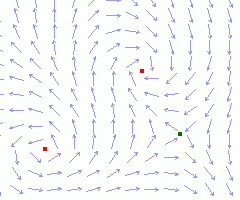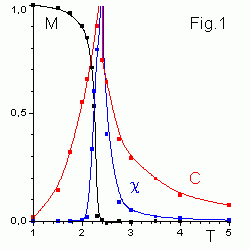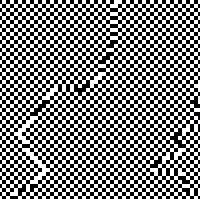it T mag   fps
512x512 Ising lattice is shown above. Up and down spins are white and black pixels (squares). You can watch thermal fluctuations, phase transition and clusters formation (or melting) for different temperatures. Drag "thermometer" to the right. The black bar corresponds to the phase transition temperature Tc. Antiferromagnetic spins ordering appears for negative temperature.
The thermostat algorithm is used. it is the iteration per frame number. mag is "magnification". Choose 1024x1024 lattice.

# Phase transitions in magneticsPhase transitions are observed in surprisingly simple systems, e.g. on a lattice of interacting spins si (magnet vectors). Interaction energy of nearest neighbours pair is
Eij = -J (si sj ) .
Total energy E and magnetization M for a spins configuration {s1, s2, ... sn } is obtained by summation throughout the lattice. In the Heisenberg model every spin can take arbitrary direction. In the XY model spins rotate in a plane. In the Ising model spins have only two possible states ±1 (up or down). As since every spin takes two values, therefore there are 2n different configurations for n spins. You see below 24 = 16 spin configurations for 2x2 lattice.
``` E = -4J            E = 4J
+ +   - -          + -   - +
+ +   - -          - +   + -

E = 0
- +   + -   + +   + +    + -   - +   - -   - -    - -   + -   + +   - +
+ +   + +   + -   - +    - -   - -   - +   + -    + +   + -   - -   - +
```For J > 0 the state of lowest energy is when all spins are aligned. The state has macroscopic magnetizaion, i.e. it is ferromagnetic. When temperature is low Ising spins minimize energy. Interaction aligns all spin vectors in the same direction, giving huge total magnetic fields. At high temperature thermal fluctuations break spins order. The randomness of the spin configuration tends to wash out the large scale magnetism. In the 2D Ising model there is a phase transition at Tc = 2.269 from disordered (non-magnetic) to ordered magnetic state (see Fig.1).

## AntiferromagneticsIf the exchange interaction constant J < 0 , then for low temperature nearest spins are anti-aligned. In the simplest Ising antiferromagnetic on square grid they form two ordered sub-lattices (disposed as cells on a chess-board). Starting at random configuration you can see clusters of ordered phase formation and movement of domain walls. Antiferromagnetic ordering appears in the thermostat algorithm for negative temperature.

# The Monte-Carlo method

For large systems it is impossible to calculate statistical averages directly. One could generate spins configurations at random and approximate real thermal averages by Monte-Carlo averages. The next problem here is, most randomly chosen states will make a negligible contribution to the sum. Random walks are used to take into account only important spins configurations by the introduction of a fictitious dynamics (so-called Markov chain of configurations). This wandering generates states, which are the most probable from energy-entropy point of view (importance sampling).

## The Metropolis algorithm

In the Metropolis algorithm we try to turn over a single spin direction with transition probability
 W12 = exp[(E1-E2)/T] if   E1 < E2 W12 = 1 if   E1 > E2
where E1, E2 are energies of the old and new configurations (see details in the Gould and Tobochnik book). Statistical averages may then be computed as simple arithmetic means. The Metropolis algorithm can by applied easy e.g. to the XY model simulation. There are many possible choices for W12. To get equilibrium final spins distribution it is enough that Wij satisfy the detailed balance conditions
W12 exp(-E1/T) = W21 exp(-E2/T) or
W12 / W21 = exp[(E1-E2)/T].

## The thermostat algorithm

In the thermostat algorithm we get a single spin (all the rest are fixed) into contact with big thermostat at temperature T. Then we get for transition probabilities (the Glauber formula)
P+ = exp(-E+ /T) / [exp(-E+ /T) + exp(-E- /T)] = exp(-(E+-E- )/T) / [exp(-(E+-E- )/T) + 1] ,
P- = exp(-E- /T) / [exp(-E+ /T) + exp(-E- /T)] = 1 - P+ .

The Glauber transition probabilities satisfy the detailed balance conditions.

In this application spins are processed in parallel on GPU (WebGL 2) in the chess-board order. The linear congruential random number generator is used
r = 1664525u * r + 1013904223u,
where r is unsigned 32 bit integer. It is initialized by 0xFFFFFFFF*Math.random() values.

Contents   Next: Potts model
updated 3 Oct 2015